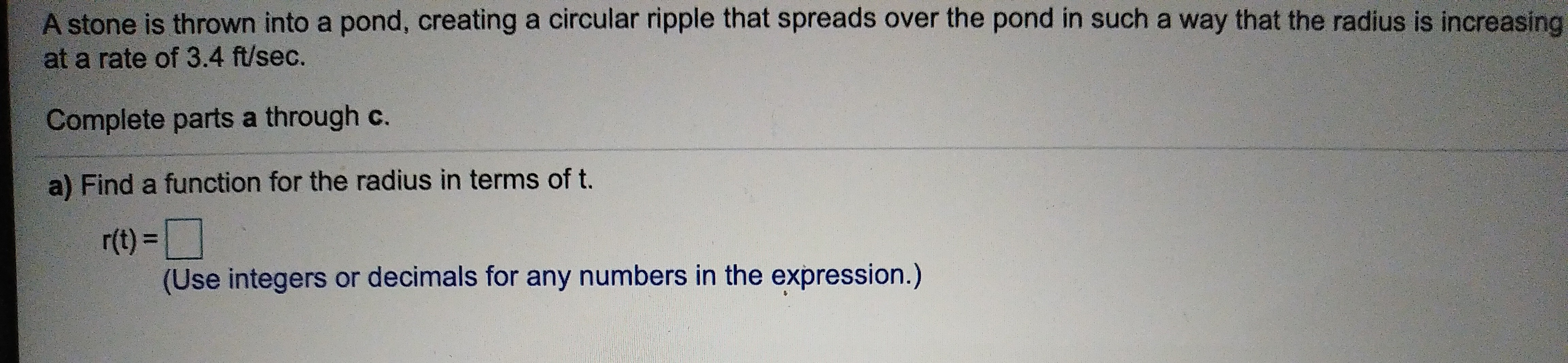# A stone is thrown into a pond, creating a circular ripple that spreads over the pond in such a way that the radius is increasingat a rate of 3.4 ft/sec.Complete parts a through c.a) Find a function for the radius in terms of t.r(t)=(Use integers or decimals for any numbers in the expression.)

Question
54 viewshelp_outlineImage TranscriptioncloseA stone is thrown into a pond, creating a circular ripple that spreads over the pond in such a way that the radius is increasing at a rate of 3.4 ft/sec. Complete parts a through c. a) Find a function for the radius in terms of t. r(t)= (Use integers or decimals for any numbers in the expression.) fullscreen
check_circle

Step 1

Given that a stone is thrown into a pond, creating a circular ripple that spreads over the pond in such a way that the radius is increasing at a rate of 3.4 ft/sec.

Step 2

Since, in one second the increment in radius is 3.4 ft. So,...

### Want to see the full answer?

See Solution

#### Want to see this answer and more?

Solutions are written by subject experts who are available 24/7. Questions are typically answered within 1 hour.*

See Solution
*Response times may vary by subject and question.
Tagged in

### Other